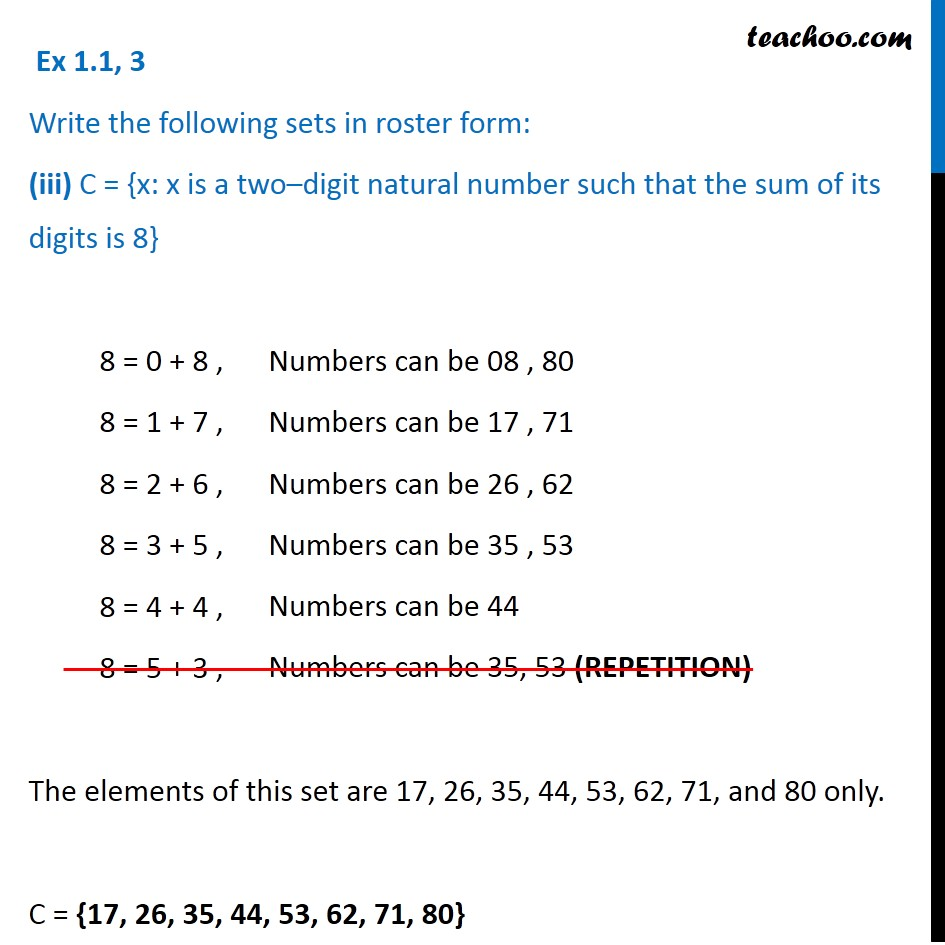Ex 1.1

Chapter 1 Class 11 Sets
Serial order wiseLearn in your speed, with individual attention - Teachoo Maths 1-on-1 Class

### Transcript

Ex 1.1, 3 Write the following sets in roster form: (iii) C = {x: x is a two–digit natural number such that the sum of its digits is 8} 8 = 0 + 8 , 8 = 1 + 7 , 8 = 2 + 6 , 8 = 3 + 5 , 8 = 4 + 4 , 8 = 5 + 3 , The elements of this set are 17, 26, 35, 44, 53, 62, 71, and 80 only. C = {17, 26, 35, 44, 53, 62, 71, 80}